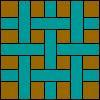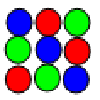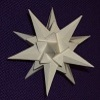Search by Topic

Resources tagged with Modular arithmetic similar to Maths Shop Window:

Filter by: Content type:
Age range:
Challenge level:

There are 38 results

Broad Topics > Numbers and the Number System > Modular arithmeticAge 16 to 18 Challenge Level:

Decipher a simple code based on the rule C=7P+17 (mod 26) where C is the code for the letter P from the alphabet. Rearrange the formula and use the inverse to decipher automatically.Double Time

Age 16 to 18 Challenge Level:

Crack this code which depends on taking pairs of letters and using two simultaneous relations and modulus arithmetic to encode the message.Modular Fractions

Age 16 to 18 Challenge Level:

We only need 7 numbers for modulus (or clock) arithmetic mod 7 including working with fractions. Explore how to divide numbers and write fractions in modulus arithemtic.The Knapsack Problem and Public Key Cryptography

Age 16 to 18

An example of a simple Public Key code, called the Knapsack Code is described in this article, alongside some information on its origins. A knowledge of modular arithmetic is useful.Check Code Sensitivity

Age 14 to 16 Challenge Level:

You are given the method used for assigning certain check codes and you have to find out if an error in a single digit can be identified.Filling the Gaps

Age 14 to 16 Challenge Level:

Which numbers can we write as a sum of square numbers?Sixinit

Age 16 to 18 Short Challenge Level:

Choose any whole number n, cube it, add 11n, and divide by 6. What do you notice?Zeller's Birthday

Age 14 to 16 Challenge Level:

What day of the week were you born on? Do you know? Here's a way to find out.Take Three from Five

Age 14 to 16 Challenge Level:

Caroline and James pick sets of five numbers. Charlie chooses three of them that add together to make a multiple of three. Can they stop him?Knapsack

Age 14 to 16 Challenge Level:

You have worked out a secret code with a friend. Every letter in the alphabet can be represented by a binary value.Modulus Arithmetic and a Solution to Dirisibly Yours

Age 16 to 18

Peter Zimmerman from Mill Hill County High School in Barnet, London gives a neat proof that: 5^(2n+1) + 11^(2n+1) + 17^(2n+1) is divisible by 33 for every non negative integer n.More Sums of Squares

Age 16 to 18

Tom writes about expressing numbers as the sums of three squares.Modulus Arithmetic and a Solution to Differences

Age 16 to 18

Peter Zimmerman, a Year 13 student at Mill Hill County High School in Barnet, London wrote this account of modulus arithmetic.Prime AP

Age 16 to 18 Challenge Level:

What can you say about the common difference of an AP where every term is prime?Transposition Fix

Age 14 to 16 Challenge Level:

Suppose an operator types a US Bank check code into a machine and transposes two adjacent digits will the machine pick up every error of this type? Does the same apply to ISBN numbers; will a machine. . . .The Chinese Remainder Theorem

Age 14 to 18

In this article we shall consider how to solve problems such as "Find all integers that leave a remainder of 1 when divided by 2, 3, and 5."Check Codes

Age 14 to 16 Challenge Level:

Details are given of how check codes are constructed (using modulus arithmetic for passports, bank accounts, credit cards, ISBN book numbers, and so on. A list of codes is given and you have to check. . . .Small Groups

Age 16 to 18

Learn about the rules for a group and the different groups of 4 elements by doing some simple puzzles.Mod 3

Age 14 to 16 Challenge Level:

Prove that if a^2+b^2 is a multiple of 3 then both a and b are multiples of 3.Mod 7

Age 16 to 18 Challenge Level:

Find the remainder when 3^{2001} is divided by 7.Remainder Hunt

Age 16 to 18 Challenge Level:

What are the possible remainders when the 100-th power of an integer is divided by 125?Odd Stones

Age 14 to 16 Challenge Level:

On a "move" a stone is removed from two of the circles and placed in the third circle. Here are five of the ways that 27 stones could be distributed.Euler's Officers

Age 14 to 16 Challenge Level:

How many different ways can you arrange the officers in a square?More Mods

Age 14 to 16 Challenge Level:

What is the units digit for the number 123^(456) ?Novemberish

Age 14 to 16 Challenge Level:

a) A four digit number (in base 10) aabb is a perfect square. Discuss ways of systematically finding this number. (b) Prove that 11^{10}-1 is divisible by 100.Elevens

Age 16 to 18 Challenge Level:

Add powers of 3 and powers of 7 and get multiples of 11.Latin Squares

Age 11 to 18

A Latin square of order n is an array of n symbols in which each symbol occurs exactly once in each row and exactly once in each column.Rational Round

Age 16 to 18 Challenge Level:

Show that there are infinitely many rational points on the unit circle and no rational points on the circle x^2+y^2=3.Guesswork

Age 14 to 16 Challenge Level:

Ask a friend to choose a number between 1 and 63. By identifying which of the six cards contains the number they are thinking of it is easy to tell them what the number is.Grid Lockout

Age 14 to 16 Challenge Level:

What remainders do you get when square numbers are divided by 4?Pythagoras Mod 5

Age 16 to 18 Challenge Level:

Prove that for every right angled triangle which has sides with integer lengths: (1) the area of the triangle is even and (2) the length of one of the sides is divisible by 5.The Public Key

Age 16 to 18 Challenge Level:

Find 180 to the power 59 (mod 391) to crack the code. To find the secret number with a calculator we work with small numbers like 59 and 391 but very big numbers are used in the real world for this.Dirisibly Yours

Age 16 to 18 Challenge Level:

Find and explain a short and neat proof that 5^(2n+1) + 11^(2n+1) + 17^(2n+1) is divisible by 33 for every non negative integer n.Obviously?

Age 14 to 18 Challenge Level:

Find the values of n for which 1^n + 8^n - 3^n - 6^n is divisible by 6.Old Nuts

Age 16 to 18 Challenge Level:

In turn 4 people throw away three nuts from a pile and hide a quarter of the remainder finally leaving a multiple of 4 nuts. How many nuts were at the start?Purr-fection

Age 16 to 18 Challenge Level:

What is the smallest perfect square that ends with the four digits 9009?Happy Birthday

Age 16 to 18 Challenge Level:

Can you interpret this algorithm to determine the day on which you were born?The Best Card Trick?

Age 11 to 16 Challenge Level:

Time for a little mathemagic! Choose any five cards from a pack and show four of them to your partner. How can they work out the fifth?